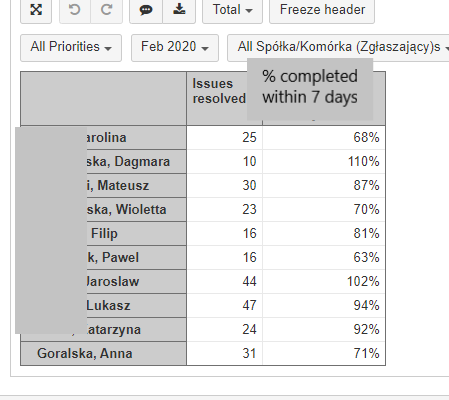# % of cases completed on time

Hi,

I need your help. I try to present all cases completed on time (within 7 business days).

To do that, I first count the number of completed cases up to 7 working days:

NonZero(
Count(
Filter(
Descendants([Issue].CurrentHierarchyMember,[Issue].[Issue]),
Not IsEmpty([Measures].[Issue resolution date])
AND
[Measures].[Issues created]>0
AND
Datediffworkdays(
DateWithoutTime([Measures].[Issue created date]),
DateWithoutTime([Measures].[Issue resolution date])
)<7
)
)
)

Then I count the percentage of cases completed within 7 days of all cases completed:

([Measures].[completed within 7 days], [assigned person].CurrentHierarchyMember)
/
([Measures].[Issues resolved], [assigned person].CurrentHierarchyMember)

Unfortunately, some people have over 100% of cases completed on time. This is due to the fact that cases started in one month and completed in the next are counted as " infinity% ".
What can I do?Hi @Kamila,

First, you can try to use the Sum() function to calculate the number of issues resolved within seven workdays. It has proven to work more efficiently than the Count() function.

The other thing, the formula looks at resolved issues, but the measure “Issues created” is used in the filter. That, in combination with the Time dimension, makes the formula count issues that were created within that month, not resolved. It accounts for some strange results like you noted. You can change that by using the measure “Issues resolved”.

See the formula below:

``````Sum(
Filter(
Descendants([Issue].CurrentHierarchyMember,[Issue].[Issue]),
Not IsEmpty([Measures].[Issue resolution date])
AND
Datediffworkdays(
DateWithoutTime([Measures].[Issue created date]),
DateWithoutTime([Measures].[Issue resolution date])
)<7
),[Measures].[Issues resolved]
)
``````

eazyBI documentation has more information on how measures work with the Time dimension - https://docs.eazybi.com/eazybijira/analyze-and-visualize/calculated-measures-and-members/calculated-members-in-time-dimension#CalculatedmembersinTimedimension-MovinginTime.

Best,
Roberts // support@eazybi.com